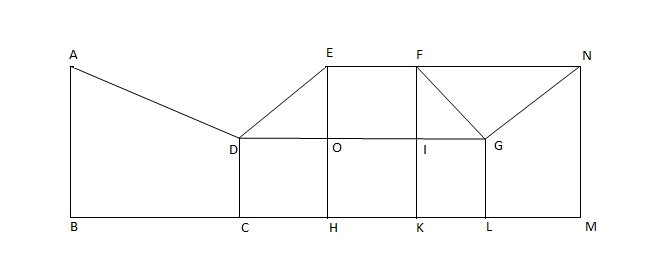### CAT 2002 Question Paper Question 5

Instructions

Directions for the next two questions: Answer the questions based on the following diagram

In the following diagram, $$\angle{ABC}$$ = 90° = $$\angle{DCH}$$ = $$\angle{DOE}$$ = $$\angle{EHK}$$ = $$\angle{FKL}$$ = $$\angle{GLM}$$ = $$\angle{LMN}$$

AB = BC = 2CH = 2CD = EH = FK = 2HK = 4KL = 2LM = MNQuestion 5

# The magnitude of $$\angle{FGO}$$ =

Solution

The length of FI is twice the length of IG.

So, the sides of the triangle FIG are in the ratio 2:1:$$\sqrt5$$.

So, angle FGO = angle FGI, which is definitely not equal to 30 or 45 or 60.

Hence, option D is the answer.